Question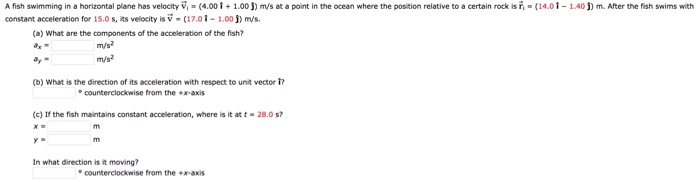We need at least 10 more requests to produce the answer.

0 / 10 have requested this problem solution

The more requests, the faster the answer.

All students who have requested the answer will be notified once they are available.

#### Earn Coins

Coins can be redeemed for fabulous gifts.

Similar Homework Help Questions
• ### Please help! A fish swimming in a horizontal plane has velocity v = (4.00 i +...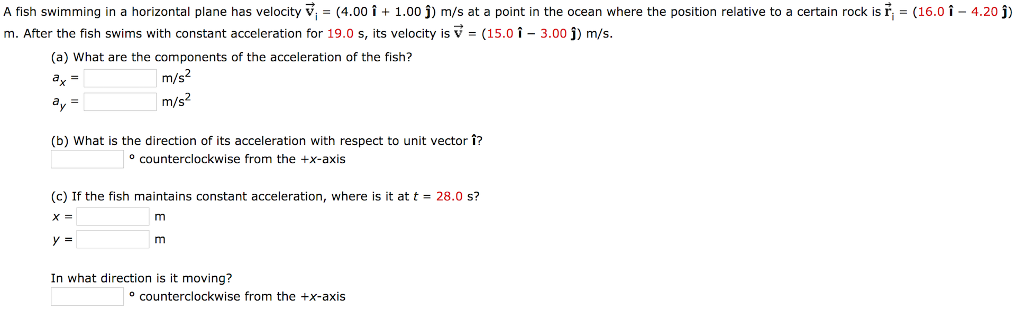Please help! A fish swimming in a horizontal plane has velocity v = (4.00 i + 1.00 j) m/s at a point in the ocean where the position relative to a certain rock is ri = (16.0-4.20 j) m. After the fish swims with constant acceleration for 19.0 s, its velocity is V (15.0 1-3.00 j) m/s. (a) What are the components of the acceleration of the fish? m/s m/s (b) What is the direction of its acceleration with respect...

• ### A fish swimming in a horizontal plane has velocity v with arrowi = (4.00 i +...

A fish swimming in a horizontal plane has velocity v with arrowi = (4.00 i + 1.00 j) m/s at a point in the ocean where the position relative to a certain rock is r with arrowi = (14.0 i − 4.20 j) m. After the fish swims with constant acceleration for 19.0 s, its velocity is v with arrow = (15.0 i − 3.00 j) m/s. (a) What are the components of the acceleration of the fish? ax =...

• ### Help! A fish swimming in a horizontal plane has velocity V (4.00 i1.00 j) m/s at...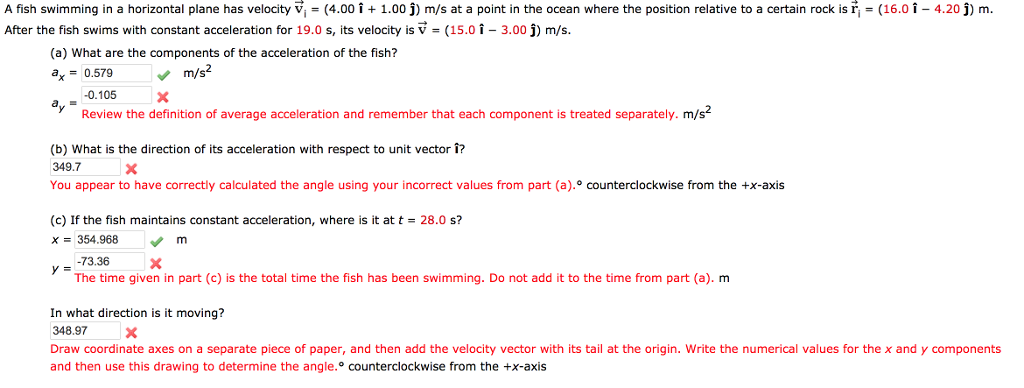Help! A fish swimming in a horizontal plane has velocity V (4.00 i1.00 j) m/s at a point in the ocean where the position relative to a certain rock is fter the fish swims with constant acceleration for 19.0 s, its velocity is V (15.0 1-3.00 j) m/s (16.0 1 4.20) m (a) What are the components of the acceleration of the fish? ax0.579 m/s2 0.105X Review the definition of average acceleration and remember that each component is treated separately....

• ### A fish swimming in a horizontal plane has velocity m/s at a point in a the...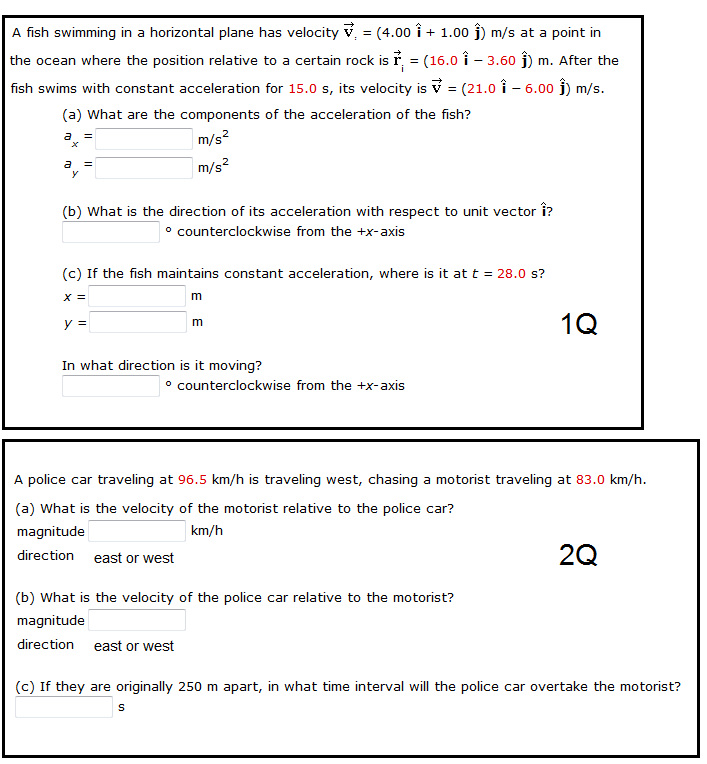A fish swimming in a horizontal plane has velocity m/s at a point in a the ocean where the position relative to a certain rock is m. After the fish swims with constant acceleration for 15.0 s, its velocity is m/s. What are the components of the acceleration of the fish? What is the direction of its acceleration with respect to unit vector ? counterclockwise from the +x-axis If the fish maintains constant acceleration, where is it at t =...

• ### A fish swimming In a horlzontal plane has velocity v, (4.00 i5.00 j) m/s at a...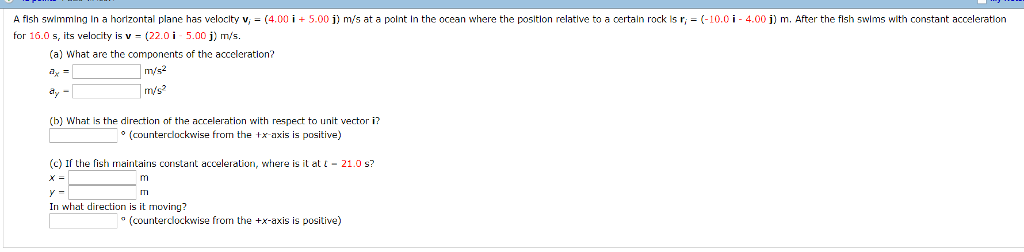A fish swimming In a horlzontal plane has velocity v, (4.00 i5.00 j) m/s at a polnt In the ocean where the positlon relatlve to a certain rock Is r(10.0 i 4.00 ) m. After the fish swlms wth contant acceleratlon for 16.0 s, its velocity is v (22.0i 5.00 j) m/s. (a) What are the components of the acceleration? ritt? (b) What is the direction of the acceleration with respect to unit vectori? (counterdlockwise from the x axis is...

• ### just the last one idk why its wrong A fish swimming in a horizontal plane has...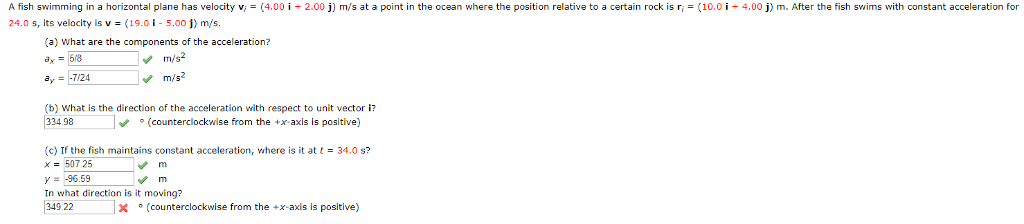just the last one idk why its wrong A fish swimming in a horizontal plane has velocity v 24.0 s, its velocity is v(19.01-5.001) m/s 4.00 і 2.00 j) m s at a point in the ocean where the position relat ve to a certan rocks 4.00 m After the ist swi snitt constan acceleration (a) What are the components of the acceleration? a,-58 m/s2 (b) what is the direction of the acceleration with respect to unit vector i 334...

• ### 5. + 0/1 points Previous Answers SerPSE 10 4.2.OP.005.MI. My Notes A fish swimming in a...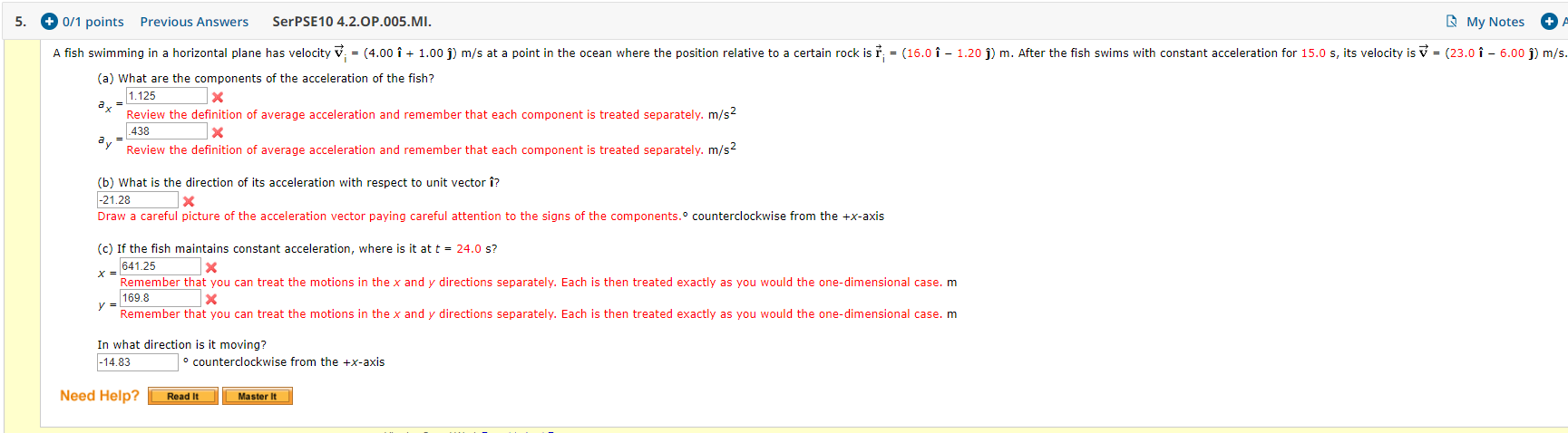5. + 0/1 points Previous Answers SerPSE 10 4.2.OP.005.MI. My Notes A fish swimming in a horizontal plane has velocity v = (4.00 i + 1.00 )) m/s at a point in the ocean where the position relative to a certain rock is † ; = (16.0 î - 1.20 j) m. After the fish swims with constant acceleration for 15.0 s, its velocity is v = (23.0 î - 6.00 ) m/s. (a) What are the components of the...

• ### Please answer all. The displacement vectors A and B shown in the figure below both have...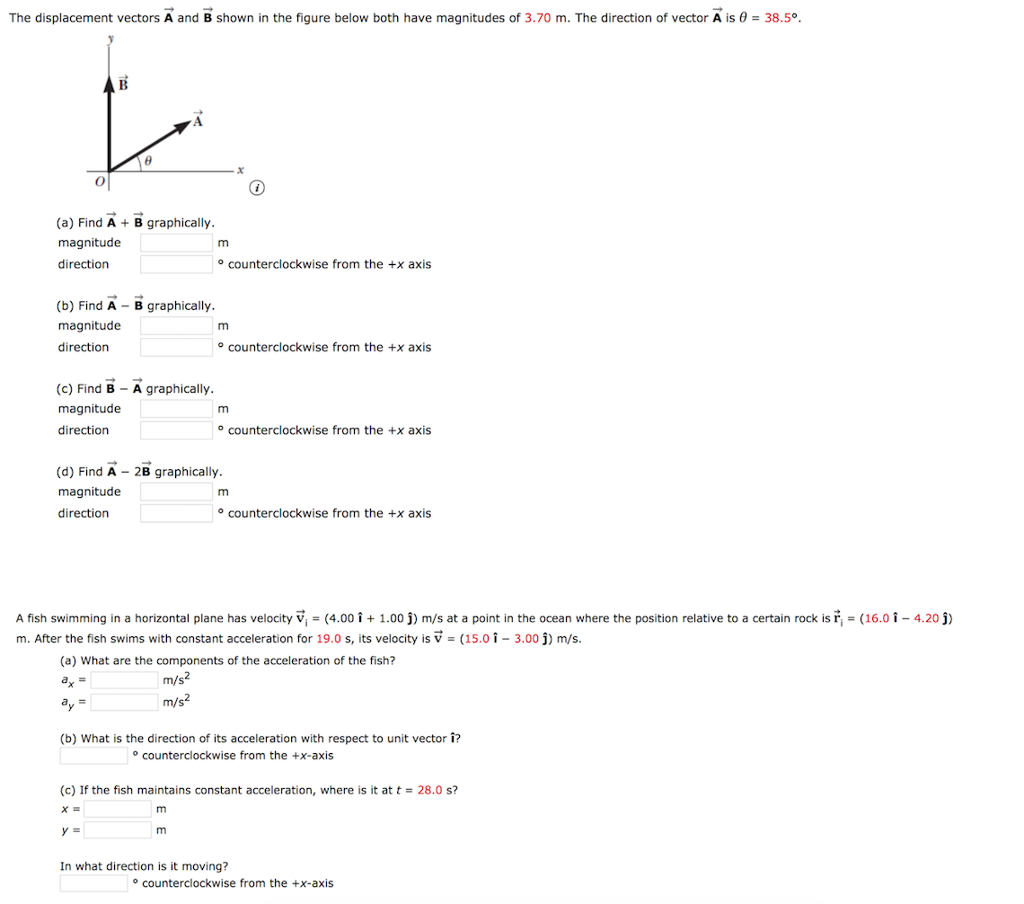Please answer all. The displacement vectors A and B shown in the figure below both have magnitudes of 3.70 m. The direction of vector A is θ = 38.5° (a) Find A B graphically magnitude direction o counterclockwise from the +x axis (b) Find A B graphically magnitude direction o counterclockwise from the +x axis (c) Find B A graphically magnitude direction o counterclockwise from the +x axis (d) Find A 2B graphically magnitude direction o counterclockwise from the +x...

• ### If the number is a decimal please include 4 digits past the decimal point if available....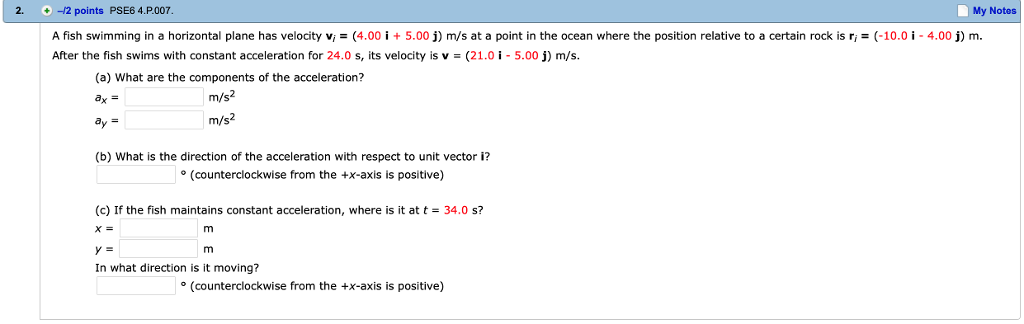If the number is a decimal please include 4 digits past the decimal point if available. 2. -12 points PSE6 4.P.007 My Notes A fish swimming in a horizontal plane has velocity vi = (4.00 İ + 5.00 j) m/s at a point in the ocean where the position relative to a certain rock is ri = (-10.01-4.00 j) m. After the fish swims with constant acceleration for 24.0 s, its velocity is v-(21.0-5.00) m/s. (a) What are the components...

• ### An object traveling along the x-axis wit h constant acceleration has velocity v = +13.0 m/s...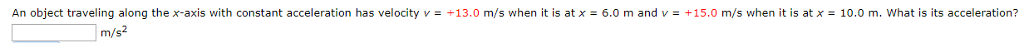An object traveling along the x-axis wit h constant acceleration has velocity v = +13.0 m/s when it is at x-6.0 m and v-+ 15.0 m/s when it is at x-10.0 m, what is its acceleration? t is at x 10.0 m. What is its acceleration? m/s